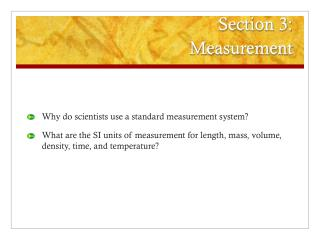DownloadDownload PresentationSection 3: Measurement

# Section 3: Measurement

Télécharger la présentation## Section 3: Measurement

- - - - - - - - - - - - - - - - - - - - - - - - - - - E N D - - - - - - - - - - - - - - - - - - - - - - - - - - -
##### Presentation Transcript

1. Section 3:Measurement • Why do scientists use a standard measurement system? • What are the SI units of measurement for length, mass, volume, density, time, and temperature?

2. A Standard Measurement System • Using SI as the standard system of measurement allows scientists to compare data and communicate with each other about their results. SI units- based on multiples of 10.

3. Length • The basic unit of length in SI is the meter (m).

4. Mass • The SI unit of mass is the kilogram. Mass refers to the amount of matter an object contains.

5. Weight • Weight refers to the measure of the force of gravity on an object. • Less gravity, less weight! • Weight is measured in Newtons (we use pounds in USA)

6. Volume • Volume is the amount of space matter takes up • The SI unit of volume is the cubic meter (m3). Volume of a regular solid is measured by multiplying length x width x height

7. Volume • Volume of irregular solids must be measured by water displacement

8. Density • The SI unit for density is kilogram per cubic meter or kg/m3 • Density =

9. Density • Because density is actually made up of two other measurements–mass and volume–an object’s density is expressed as a combination of two units. The density of a substance stays the same no matter how large or small a sample of the substance is.

10. Time • The SI unit of time is seconds. Some timepieces can measure within one one hundredth of a second!

11. Temperature • The SI unit for temperature is Celsius or Kelvin scale • 0 K is called absolute zero and is equal to -273C

12. Temperature Scientists use the Celsius and Kelvin scales to measure temperature. The Kelvin (K) is the SI unit of temperature. We will use Celsius!

13. Water freezes at 0 degrees Celsius and boils at 100

14. End of Section:Measurement